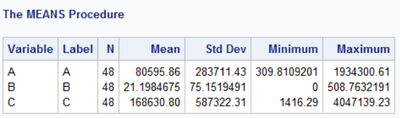## PROC IMPORT numeric truncating problem

I have attached a sample EXCEL file that consists of 3 columns with all numeric, and a 2nd column is a trouble.

``````proc import datafile = "C:\test.xls"
out = _null11111_ replace dbms = excel ;

getnames = no;
range = "sheet\$A6:c53";
dbdsopts="dbsastype=(F2='numeric')";

run;``````

After running as above code, I can find that something wrong with 2nd column.

Only 2nd column is imported as integer number. I don't know why.

The odd thing is that when I run SAS with opening Excel file, SAS can import all columns correctly.

Of course, I know that I can handle this problem by editing Excel file, but I would like to fix it with no editing Excel file.

1 ACCEPTED SOLUTION

Accepted Solutions

## Re: PROC IMPORT numeric truncating problem

Since you must have access to a copy of Excel to use the DBMS=EXCEL option in PROC IMPORT use it to save the file as an XLSX file and IMPORT the new file using the XLSX engine instead.

```1047  proc import file="~/test/test.xlsx" dbms=xlsx out=test replace;
1048  getnames = no;
1049  range = "sheet\$A6:c53";
1050  run;

NOTE: The import data set has 48 observations and 3 variables.
NOTE: WORK.TEST data set was successfully created.
NOTE: PROCEDURE IMPORT used (Total process time):
real time           0.13 seconds
cpu time            0.02 seconds

1051  data _null_;
1052  set test;
1053  if b ne int(b) then do;
1054    put (_n_ _all_) (=);
1055    stop;
1056  end;
1057  run;

_N_=1 A=8701.4623591 B=5.2105 C=21718.04648
NOTE: There were 1 observations read from the data set WORK.TEST.```

7 REPLIES 7

## Re: PROC IMPORT numeric truncating problem

I am actually more interested in the fact that PROC IMPORT allows you to use OUT=_NULL_.

How could that possibly work?

## Re: PROC IMPORT numeric truncating problem

Sorry, it's my mistake. I just roughly did it. As you said, it donesn't work with _null_ name. So I've corrected it

## Re: PROC IMPORT numeric truncating problem

What is your actual problem?  Why do you think you need to use DBSASTYPE option?

SAS can read now Excel files directly without using Microsoft software.

But I could not get SAS to read that XLS file. But if I opened it with Excel and saved it as an XLSX file then SAS can read it.

```1     proc import file="c:\downloads\test.xls" dbms=xls out=test replace;
2     run;

Memory request error
ERROR: Not enough memory available to allocate storage.
NOTE: The SAS System stopped processing this step because of errors.
NOTE: PROCEDURE IMPORT used (Total process time):
real time           0.10 seconds
cpu time            0.00 seconds

4     run;

NOTE: One or more variables were converted because the data type is not supported by the V9 engine. For
more details, run with options MSGLEVEL=I.
NOTE: The import data set has 52 observations and 4 variables.
NOTE: WORK.TEST data set was successfully created.
NOTE: PROCEDURE IMPORT used (Total process time):
real time           0.17 seconds
cpu time            0.04 seconds

6     getnames = no;
7     range = "sheet\$A6:c53";
8     run;

NOTE: The import data set has 48 observations and 3 variables.
NOTE: WORK.TEST data set was successfully created.
NOTE: PROCEDURE IMPORT used (Total process time):
real time           0.01 seconds
cpu time            0.01 seconds
```## Re: PROC IMPORT numeric truncating problem

It's always the same problem. Excel files (especially the older .xls format) are of no use for data transfer.

Save your Excel data to a delimited text file and read that with a data step. This way you have total control over what gets read into SAS, and attributes like types, formats, lengths.

## Re: PROC IMPORT numeric truncating problem

@choeb wrote:

I have attached a sample EXCEL file that consists of 3 columns with all numeric, and a 2nd column is a trouble.

``````proc import datafile = "C:\test.xls"
out = _null11111_ replace dbms = excel ;

getnames = no;
range = "sheet\$A6:c53";
dbdsopts="dbsastype=(F2='numeric')";

run;``````

After running as above code, I can find that something wrong with 2nd column.

Only 2nd column is imported as integer number. I don't know why.

The odd thing is that when I run SAS with opening Excel file, SAS can import all columns correctly.

Of course, I know that I can handle this problem by editing Excel file, but I would like to fix it with no editing Excel file.

Please describe the actual problem. Is column 2 not supposed to be numeric? Not supposed to be integer or what?

SAS does not have an "integer" data type. SAS does assign a display format, typically BEST12. or similar for numeric values. If there is no decimal component to the value then the BEST format does not show them. If you want to see more decimals, or force decimals then you assign a format that displays the values as needed. The internal values SAS stores do not change until you do something to them explicitly.

## Re: PROC IMPORT numeric truncating problem

The problem is that column 2 is imported as integer by SAS though this is actually real number with decimals

Column 2 is supposed to be numeric with decimals, but I don't know how to do it

## Re: PROC IMPORT numeric truncating problem

Since you must have access to a copy of Excel to use the DBMS=EXCEL option in PROC IMPORT use it to save the file as an XLSX file and IMPORT the new file using the XLSX engine instead.

```1047  proc import file="~/test/test.xlsx" dbms=xlsx out=test replace;
1048  getnames = no;
1049  range = "sheet\$A6:c53";
1050  run;

NOTE: The import data set has 48 observations and 3 variables.
NOTE: WORK.TEST data set was successfully created.
NOTE: PROCEDURE IMPORT used (Total process time):
real time           0.13 seconds
cpu time            0.02 seconds

1051  data _null_;
1052  set test;
1053  if b ne int(b) then do;
1054    put (_n_ _all_) (=);
1055    stop;
1056  end;
1057  run;

_N_=1 A=8701.4623591 B=5.2105 C=21718.04648
NOTE: There were 1 observations read from the data set WORK.TEST.```

Discussion stats
• 7 replies
• 3073 views
• 0 likes
• 4 in conversation